## 4.4 Expressions

(1)
An expression is a formula that defines the computation or retrieval of a value. In this International Standard, the term ``expression'' refers to a construct of the syntactic category expression or of any of the other five syntactic categories defined below.
##### Syntax
```(2)
expression ::=
relation {and relation} | relation {and then relation}
| relation {or relation}  | relation {or else relation}
| relation {xor relation}
(3)
relation ::=
simple_expression [relational_operator simple_expression]
| simple_expression [not] in range
| simple_expression [not] in subtype_mark
(4)
(5)
term ::= factor {multiplying_operator factor}
(6)
factor ::= primary [** primary] | abs primary | not primary
(7)
primary ::=
numeric_literal | null | string_literal | aggregate
| name | qualified_expression | allocator | (expression)
```
##### Name Resolution Rules
(8)
A name used as a primary shall resolve to denote an object or a value.
##### Static Semantics
(9)
Each expression has a type; it specifies the computation or retrieval of a value of that type.
##### Dynamic Semantics
(10)
The value of a primary that is a name denoting an object is the value of the object.
##### Implementation Permissions
(11)
For the evaluation of a primary that is a name denoting an object of an unconstrained numeric subtype, if the value of the object is outside the base range of its type, the implementation may either raise Constraint_Error or return the value of the object.
##### Examples
(12)
Examples of primaries:
```(13)
4.0                --  real literal
Pi                 --  named number
(1 .. 10 => 0)     --  array aggregate
Sum                --  variable
Integer'Last       --  attribute
Sine(X)            --  function call
Color'(Blue)       --  qualified expression
Real(M*N)          --  conversion
(Line_Count + 10)  --  parenthesized expression
```
(14)
Examples of expressions:
```(15)
Volume                      -- primary
not Destroyed               -- factor
2*Line_Count                -- term
-4.0                        -- simple expression
-4.0 + A                    -- simple expression
B**2 - 4.0*A*C              -- simple expression
Password(1 .. 3) = "Bwv"    -- relation
Count in Small_Int          -- relation
Count not in Small_Int      -- relation
Index = 0 or Item_Hit       -- expression
(Cold and Sunny) or Warm    -- expression (parentheses are required)
A**(B**C)                   -- expression (parentheses are required)
```

####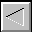|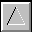|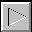|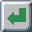|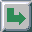TOC -- / --.-- / --.--.-- | Index | Search | Syntax | Help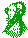-- Email comments, additions, corrections, gripes, kudos, etc. to:

Magnus Kempe -- Magnus.Kempe@di.epfl.ch